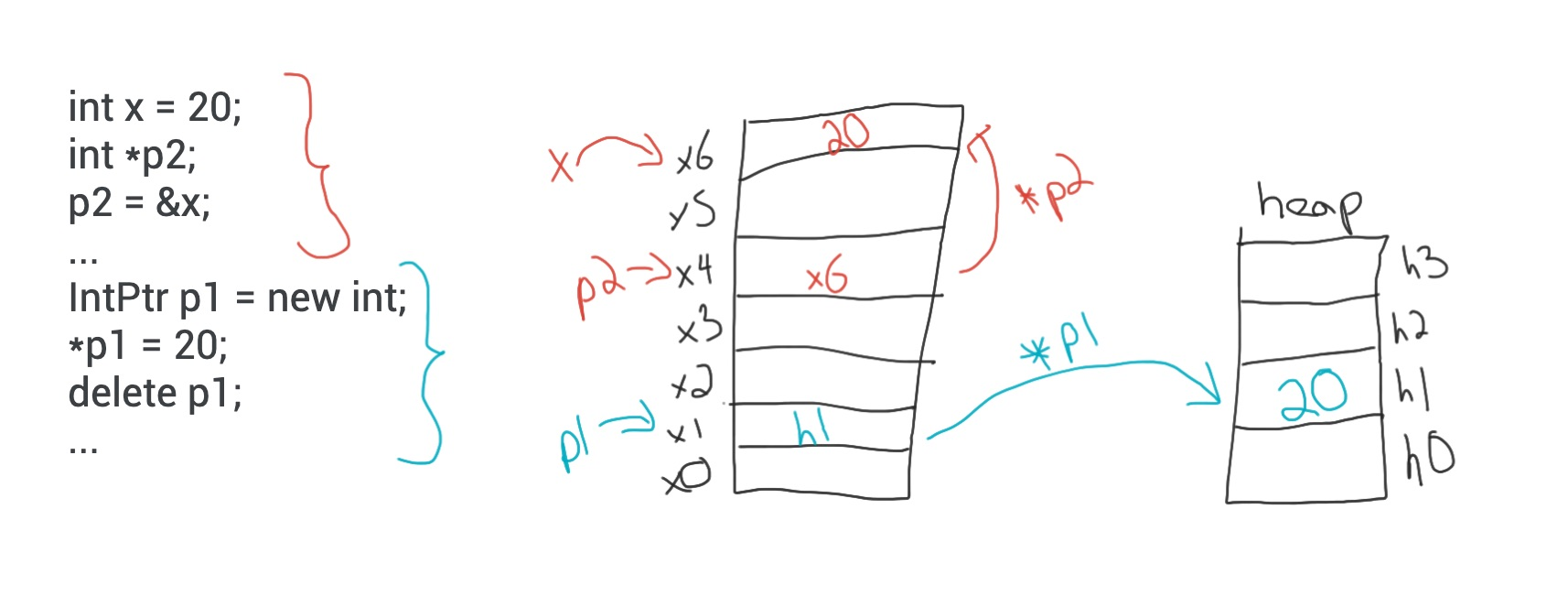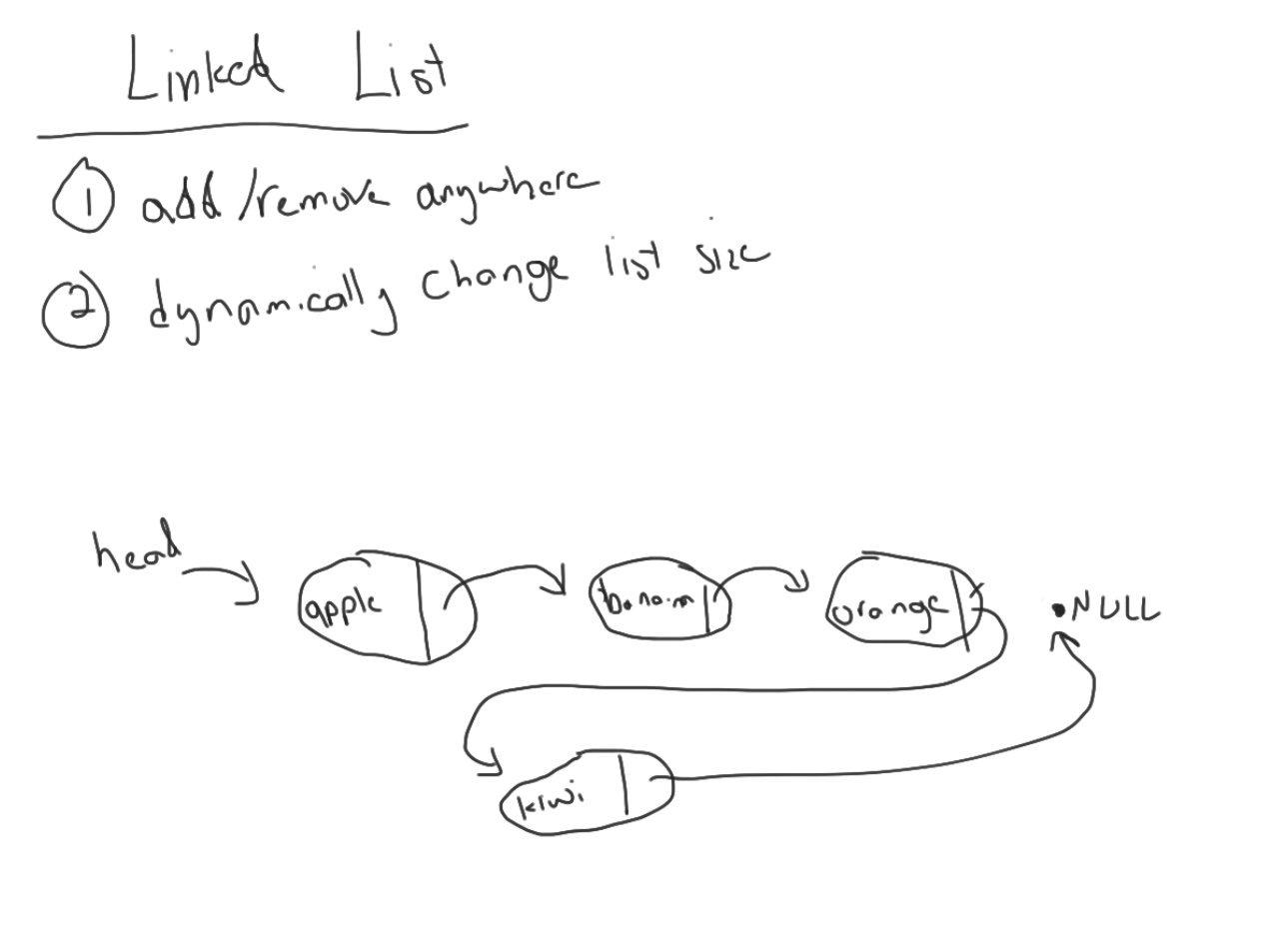# Lectures

### Lecture 1: Introduction to C++

• A first introduction to programming in C++. We will learn how to compile and run C++ programs from the command-line in Linux.
• Slides: [PDF]
• Video: No video available
• Exercises:
• References:

### LECTURE 2: Data Types, Variables & Type Casting

• Today we will learn the basics of writing C++ programs including input/output, data types and variables and type casting.
• Slides: [PDF]
• Exercises:

### LECTURE 3: BRANCHING

• Today we will learn about branching in C++ including if statements and switch statements.
• Slides: [PDF]
• Exercises:
• int_swap.cpp – in-class challenge problem to swap two integers without using a third temporary variable.
• branching_ex1.cpp – if statement example with optional else if  and else clauses.
• branching_ex2.cpp – switch statement example.

### LECTURE 4: LOOPING

• Today we will learn about looping in C++ including for loops, while loops and do-while loops.
• Slides: [PDF]
• Exercises:
• loop_ex1.cpp – three different for loop examples for adding up even numbers.
• loop_ex2.cpp – a while loop example for adding up even numbers.
• loop_ex3.cpp – a while loop example for finding the largest positive number less than 200,000 and divisible by 63.
• loop_ex4.cpp – a do-while loop example for summing positive numbers until a zero or negative number is entered by the user.

### LECTURE 7: FILE INPUT/OUTPUT I

• Today we’ll learn about file input and output using streams. How to write to files, read from a file and append to a file. Our file input and output example will also introduce arrays. Although arrays are not the main topic of the lecture they are an important programming language concept. Next, we’ll learn how to handle when a file fails to open. To assist with the example we will learn about the the chmod Linux command.
• Exercises:

### LECTURE 8: FILE INPUT/OUTPUT II

• Today we’ll learn more about file input and output using streams including how to append to a file. Next, we’ll learn how to handle when a file fails to open. To assist with the example we will learn about the the chmod Linux command.
• Notes: [chmod.txt]
• Exercises:
• References:

### LECTURE 9: CODE STYLE & DOCUMENTATION

• Overview of how to properly document your programs including in-program documentation and API documentation. We will also learn about code style – best practices for how to write and organize your programs. Examples of code style include best practices for indentation, white space, line length, comments, naming conventions and file structure.
• Slides: [PDF]
• References:

### LECTURE 10: NAMESPACES

• Overview of the four kinds of namespaces in C++: the standard namespace, user-defined namespaces, the unnamed namespace and the global namespace.
• Slides: [PDF]
• Exercises:
• namespace_ex1.cpp – examples of using the standard namespace, user-defined namespaces and unnamed namespace as well as an examples of using the scope resolution operator (::) with namespaces.

### LECTURE 11: PROBLEM SOLVING IN C++ I (CONNECT 4)

• Today we’ll build on the C++ concepts that we’ve covered in Lectures 1-10 and put them all together to program Connect 4.
• Exercises:

### LECTURE 12: PROBLEM SOLVING IN C++ II (CONNECT 4)

• Today we’ll continue to build on the C++ concepts that we’ve covered in Lectures 1-10 and put them all together to program Connect 4.
• Exercises:

### LECTURE 13: POINTERS IN C++ I

• Introduction to pointers, declaring a pointer variable, the dereferencing operator and the address-of operator.
• Slides: [PDF]

### LECTURE 14: POINTERS IN C++ II

• More pointers and defining pointer types.
• Slides: [PDF]
• Exercises:
• pointers_ex1.cpp – an example of using pointers with ordinary (automatic) variables in C++.  [YouTube]
• pointers_ex2.cpp, pointers_ex3.cpp – an example of how to pass a pointer variable to a function as a call-by-value parameter. This is the first of two examples that highlight the difference between passing a pointer as a call-by-value vs. call-by-reference parameter.  [YouTube & YouTube]

### LECTURE 15: DYNAMIC VARIABLES

• Using pointers to create dynamic variables and dynamic arrays.
• Slides: [PDF]
• Jamboard:
• Dynamic variable example:• Exercises:

### LECTURE 17: CLASSES IN C++ I

• An introduction to structures and classes in C++. We will learn about member variables, member functions including constructors, destructors, accessor and mutator functions.
• Slides: [PDF]
• Exercises:

### LECTURE 19: Implementing A STACK in C++

• Implementing a Stack class in C++ using pointers.
• Jamboard:
• Data structures:• Exercises:
• stack.cpp – a Stack class with a constructor, deconstructor, pop function, push function, isEmpty function and toString function

### LECTURE 20: Implementing A Linked List in C++

• Implementing a Linked List class in C++ using pointers.
• Jamboard: